×#### Thank you for registering.

One of our academic counsellors will contact you within 1 working day.

Click to Chat

1800-1023-196

+91-120-4616500

CART 0

• 0

MY CART (5)

Use Coupon: CART20 and get 20% off on all online Study Material

ITEM
DETAILS
MRP
DISCOUNT
FINAL PRICE
Total Price: Rs.

There are no items in this cart.
Continue Shopping• Complete Physics Course - Class 11
• OFFERED PRICE: Rs. 2,968
• View Details

```Forces between Multiple Charges

Table of Content

Forces between Multiple Charges

Principle of Superposition

General Algorithm to solve problems involving multiple charges

Forces between Multiple Charges

With the help of Coulomb’s Law, we can easily find out the mutual force of attraction/ repulsion between two charges easily. But the arising question is what to do when we have to deal with more than two charges?  Suppose in a system there are ‘n’ charges namely q1, q2, q3…qn and we are asked to find force on q1 due to other charges q2, q3….. qn. Can we use Coulomb’s Law in this case? The answer is partial yes because we know a limitation of coulomb’s law is it can’t be used directly to find force between multiple charges.

So in order to calculate force due to multiple charges, we will use Coulomb’s law with a different approach. Since force is a vector quantity, it has both direction and magnitude; it can’t be added like scalars. Addition and subtraction of vector quantity are a quite different from those of scalar quantities. Suppose mass of body A is 5 kg and mass of body B is 10 kg, then the total mass of A and B, that is, addition of A and B will yield 15 kg as the final result. Scalars involve simple algebraic additions.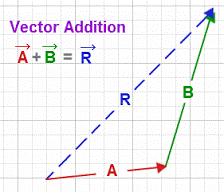Image 1: Rule of vector addition

Now let’s say there are two vectors, namely A and B, with magnitude 3 and 4 and direction in the x- direction and y-direction. Mathematically

A = 3 i

B = 4 j

Then vector sum of vector quantities A and B will be adding squares of A and B and square rooting the sum of both the squares, that is 32 + 42 = 25, and the square root of 25 is 5. So the conclusion is the vector sum of vectors A and B is 5.

So, to calculate the force between multiple charges, we can do the vector addition of all forces acting on a specific charge by other charges in a system.

Principle of Superposition

It has been experimentally verified that force on any charge due to a number of other charges is the vector sum of all the forces on that charge due to other charges are taken at a time. The individual forces remain unaffected due to other charges presence. This method is called the principle of superposition.

The principal of superposition relies on Coulomb’s statement ‘like charges repel each other and unlike charges attract each other” and can be used to find the force on any charge in a system of multiple charges.

To better understand what principle of superposition is, consider a system having charges q1, q2, q3 and q4, and we have to find the force on q1, due to other remaining three charges.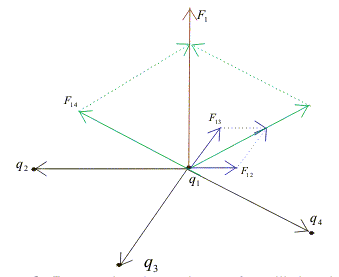Image 2: Force due to system of multiple charges

From the figure, we can see that charge q1 , faces three repulsive forces from q2 , q3 and q4 respectively. Let the force on charge q1, due to charge q2 be F12, the force on charge q1 due to charge q3 be F13 and force on charge q1 due to charge q4 be F14. And the distance between charge q1 and q2  is r12, the distance between charge q1 and q2, and the distance between charge q1 and q4 is r14.

The resultant force F will be the vector sum of F12 , F13 and F14. Mathematically

F = F12 + F13 + F14

where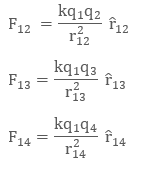Then total force on charge q1 due to other charges will be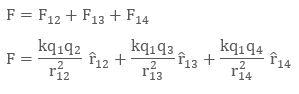Similarly, if there are ‘n’ charges in a system namely q1, q2 , q3 ……. qn  then force q1 due to other charges will be given as:Image 3: Finding net force on a specific charge if multiple charges are present in a system

Example:

Four equal charges q are kept on corners of a square, find net force on one of the charge. The side of square is ‘r’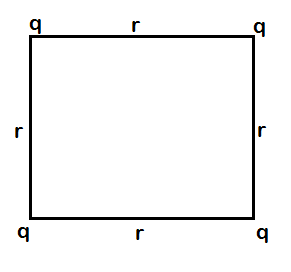Image 4: Four charges placed at corners of the square

In this problem we have four equal charges ‘q’ and distance between them is r.

Now we will take individual repulsive forces on the corner charge q and make a vector diagram and note down the forces, which as:Image 5: Vector Diagram(Force due to opposite corner charge q, which r √2  apart)

Let the resultant force on charge q be Fnet , then mathematically Fnet will be the vector sum of F, F, and F’.

Fnet = F + F + F’

The vector sum of F and F will be √2 F, then Fnet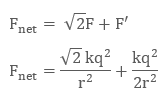General Algorithm to solve problems involving multiple charges

To make complex physics problem simple, we can follow a general algorithm:

Understand the question, analyze on which charge we have to find the net force

Make a vector diagram of all forces being repulsive/ attractive acting on the charge

Solve the vector diagram using vector addition

Watch this Video for more reference

Forces between Multiple Charges
```### Course Features

• 101 Video Lectures
• Revision Notes
• Previous Year Papers
• Mind Map
• Study Planner
• NCERT Solutions
• Discussion Forum
• Test paper with Video Solution